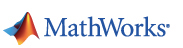# CMPSCI 689: Machine Learning, Spring 2015

## p0: Intro to MATLAB and EDLAB

### Step 1. Obtain MATLAB

For all your programming projects you will need access to MATLAB. It is simple to learn if you already know Java,C++ or Python.

First you need to get hold of a working copy of MATLAB. You may either:

• Get it via your lab (one of your machines may have it).
• Purchase a student copy from MathWorks. Get the MATLAB suite which costs 99\$ and includes various toolboxes. The image processing toolbox is particularly useful when dealing with images.
• Access MATLAB via EDLAB by remotely logging in to the instructional machines. More information on how to create an account can be found here.

### Step 2. Learn basic programming in MATLAB

MATLAB is great for prototyping and visualization which is why we choose it. There is a free alternative to MATLAB called Octave which is similar and may be used instead. However, I can't guarantee that the starter code will work with Octave. Here are some useful resources for getting started with MATLAB: